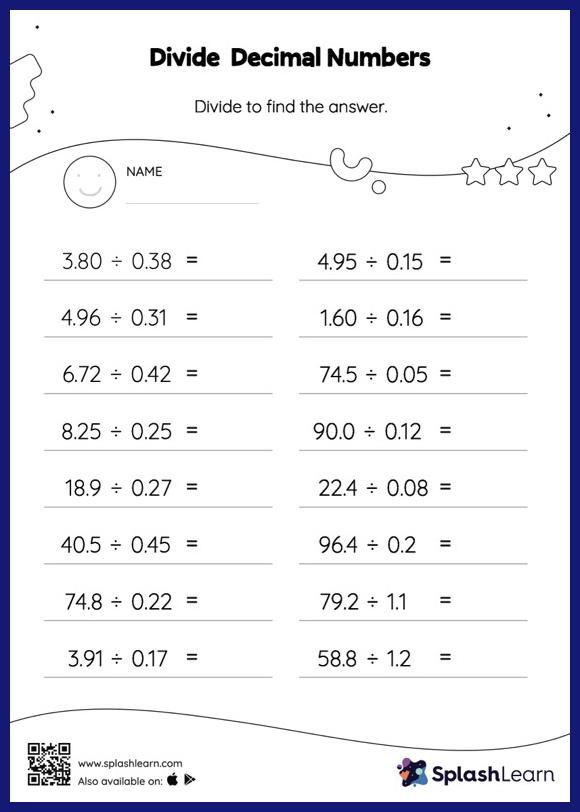# Divide Decimal Numbers without Remainder: Horizontal Division Worksheet

Home > Divide Decimal Numbers without Remainder: Horizontal DivisionThis worksheet invites students to divide decimal numbers without remainder, which helps them become proficient in division. Students first divide as though there is no decimal when dividing two decimals. They then add a decimal point at the correct location in the quotient depending on the number of decimal digits in each number. This divide decimal numbers without remainder worksheet gives students a thorough practice of this concept. In this worksheet, students practice solving problems written in the horizontal format. How numbers are laid out in a problem affects the method a student employs to solve it. Therefore to develop actual fluency and mastery of multiple strategies, students must practice different formats.